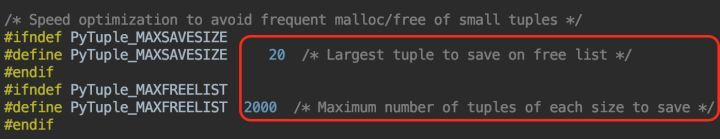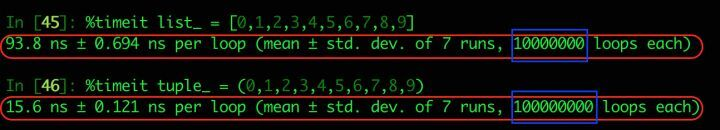# 浅析 Python 中的列表和元组

huatechinfo

0收藏1. 列表是动态数组，它们可变且可以重设长度(改变其内部元素的个数)。
2. 元组是静态数组，它们不可变，且其内部数据一旦创建便无法改变。
3. 元组缓存于Python运行时环境，这意味着我们每次使用元组时无须访问内核去 分配内存。

``new_allocated = (size_t)newsize + (newsize >> 3) + (newsize < 9 ? 3 : 6);``

``````static PyObject *
tupleconcat(PyTupleObject *a, PyObject *bb)
{
...
size = Py_SIZE(a) + Py_SIZE(b);
np = tuple_alloc(size);
...
return (PyObject *)np;

}
static PyObject *
tuplerepeat(PyTupleObject *a, Py_ssize_t n)
{
...
size = Py_SIZE(a) * n;
np = tuple_alloc(size);
...

return (PyObject *) np;
}``````帖子
视频
声望
粉丝
社区精华内容